# NCERT Solutions for Class 9 Science Work and Energy part 1

NCERT solutions for Science Work and Energy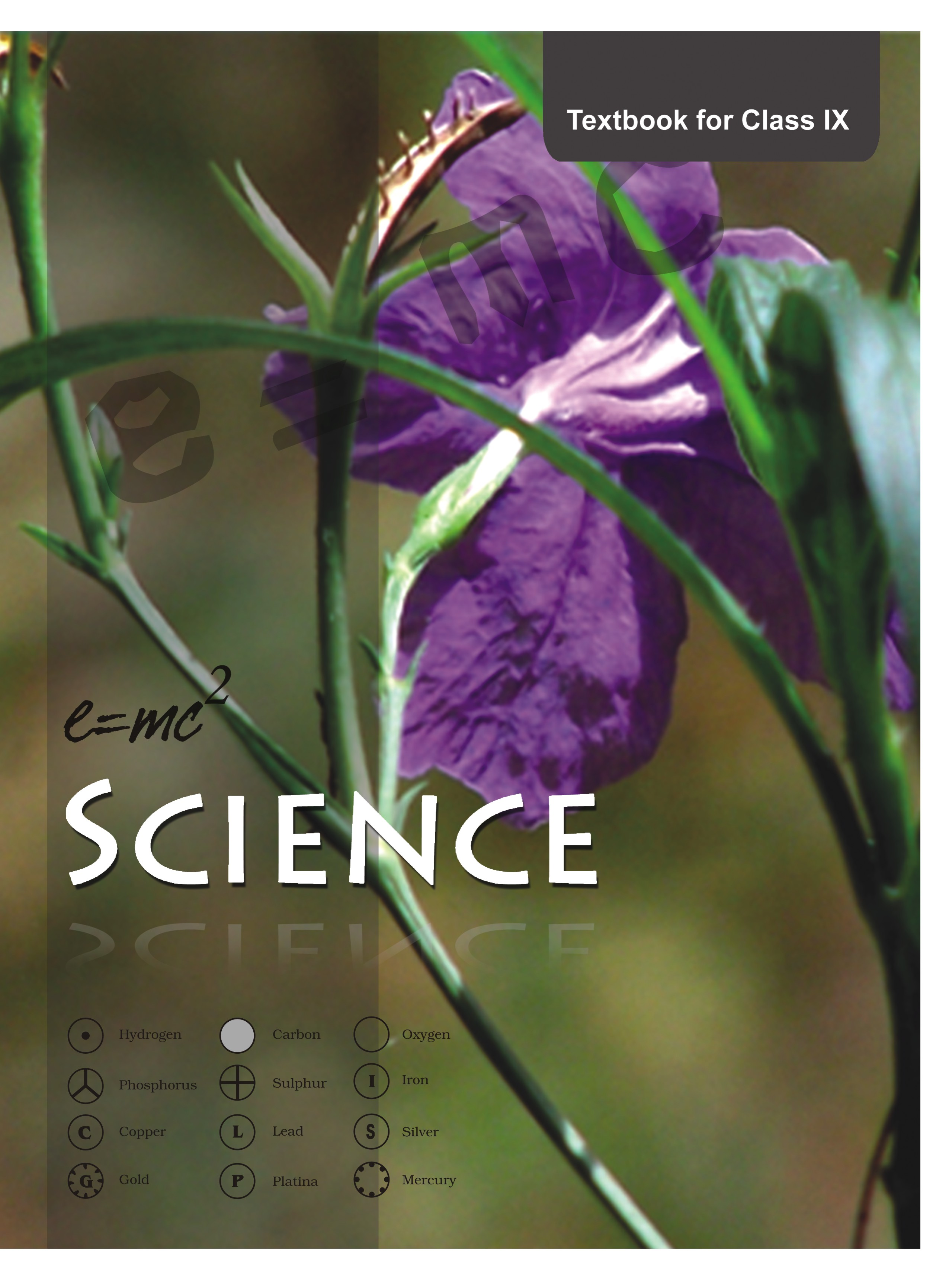## NCERT Class 9 Science Chapter wise Solutions

• 01 –  Matter in Our Surroundings
• 02 – Is Matter Around us Pure
• 03 – Atoms and Molecules
• 04 – Structure of the Atom
• 05 – The Fundamental Unit of Life
• 06 – Tissues
• 07 – Diversity in Living Organisms
• 08 – Motion
• 09 – Force and Laws of Motion
• 10 – Gravitation
• 11 – Word and Energy
• 12 – Sound
• 13 – Why Do We Fall Ill
• 14 – Natural Resources
• 15 – Improvement in Food Resources

## NCERT Solutions for Class 9 Science Work and Energy part 1

###### 1. A force of 7 N acts on an object. The displacement is, say 8 m, in the direction of the force. Let us take it that the force acts on the object through the displacement. What is the work done in this case?

Ans. Since Work (W)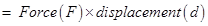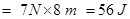NCERT Solutions for Class 9 Science Work and Energy part 1

###### 1. When do we say that work is done?

Ans. Work is done when a force acts on an object and displacement occurs in the direction of force.

###### 2. Write an expression for the work done when a force is acting on an object in the direction of its displacement.

Ans.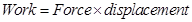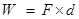###### 3. Define 1 J of work.

Ans. 1J is that work which is done when a force of 1N is applied on an object and it moves a distance of 1m in the direction of force.

###### 4. A pair of bullocks exerts a force of 140 N on a plough. The field being ploughed is 15 m long. How much work is done in ploughing the length of the field?

Ans. Work done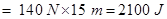NCERT Solutions for Class 9 Science Work and Energy part 1

###### 1. What is the kinetic energy of an object?

Ans. Kinetic energy of an object is a kind of mechanical energy and it is present in that object due to its state of motion(movement).

###### 2. Write an expression for the kinetic energy of an object.

Ans. Kinetic energy =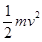where, m = mass of the object, v = velocity of the object(motion)

NCERT Solutions for Class 9 Science Work and Energy part 1

###### 3. The kinetic energy of an object of mass, m moving with a velocity of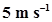is 25 J. What will be its kinetic energy when its velocity is doubled? What will be its kinetic energy when its velocity is increased three times?

Ans. K.E. of the object =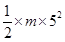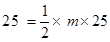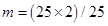= 2 kg

If velocity is doubled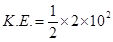= 200/2 = 100 J i.e. K.E. will become four times

If velocity is increased three times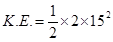= 225 J i.e. K.E. will become nine times

NCERT Solutions for Class 9 Science Work and Energy part 1

###### 1. What is power?

Ans. 1. Power is the rate of work done. It is denoted by P.

power = work/time

P = W / t

###### 2. Define 1 watt of power.

Ans. If an agent works at the rate of 1 J/s then the power of that agent is 1 watt (where watt is the unit of power).

###### 3. A lamp consumes 1000 J of electrical energy in 10 s. What is its power?

Ans. P = W/t = 1000J/10s = 100 W

NCERT Solutions for Class 9 Science Work and Energy part 1

###### 4. Define average power.

Ans. An agent may not be able to perform same amount of work in a given period of time always. In other words, power of that agent may vary with time. Hence we can take average power in such situations. Average power is defined as average amount of work done by a body per unit time (i.e. total energy consumed divided by total time).

## NCERT Solutions for Class 9 Science

NCERT Solutions Class 9 Science PDF (Download) Free from myCBSEguide app and myCBSEguide website. Ncert solution class 9 Science includes text book solutions .NCERT Solutions for CBSE Class 9 Science have total 15 chapters. 9 Science NCERT Solutions in PDF for free Download on our website. Ncert Science class 9 solutions PDF and Science ncert class 9 PDF solutions with latest modifications and as per the latest CBSE syllabus are only available in myCBSEguide.

## CBSE app for Class 9

To download NCERT Solutions for class 9 Science, Computer Science, Home Science,Hindi ,English, Maths Social Science do check myCBSEguide app or website. myCBSEguide provides sample papers with solution, test papers for chapter-wise practice, NCERT solutions, NCERT Exemplar solutions, quick revision notes for ready reference, CBSE guess papers and CBSE important question papers. Sample Paper all are made available through the best app for CBSE students and myCBSEguide website.### Test Generator

Create question paper PDF and online tests with your own name & logo in minutes.### myCBSEguide

Question Bank, Mock Tests, Exam Papers, NCERT Solutions, Sample Papers, Notes